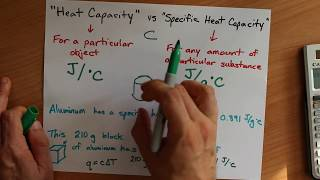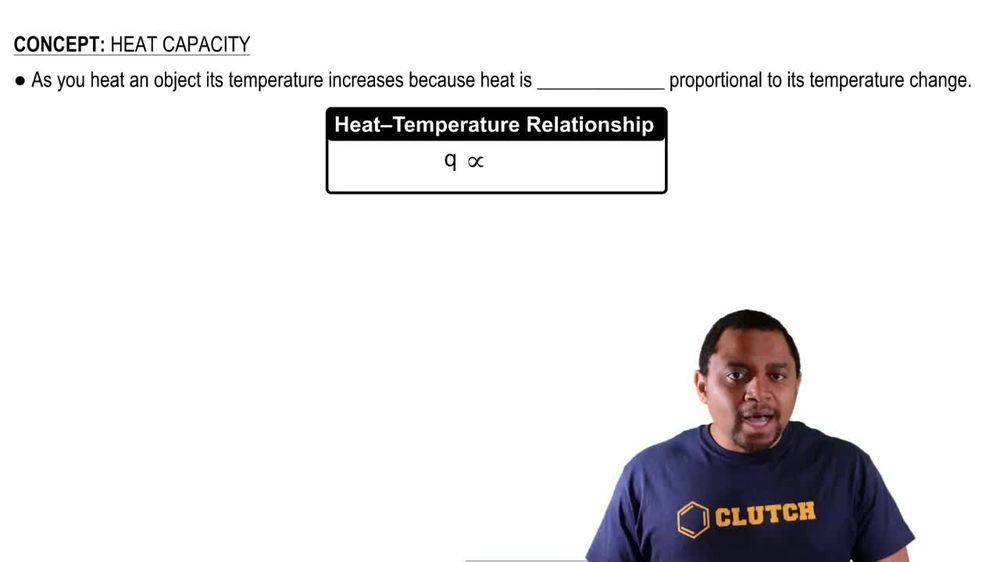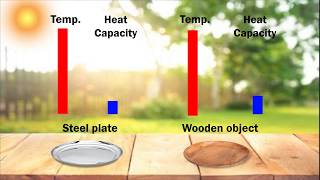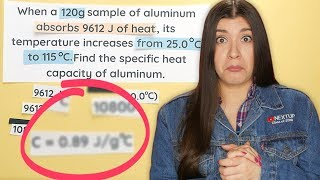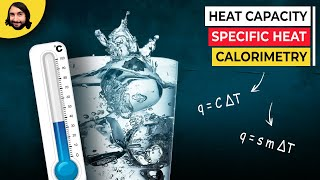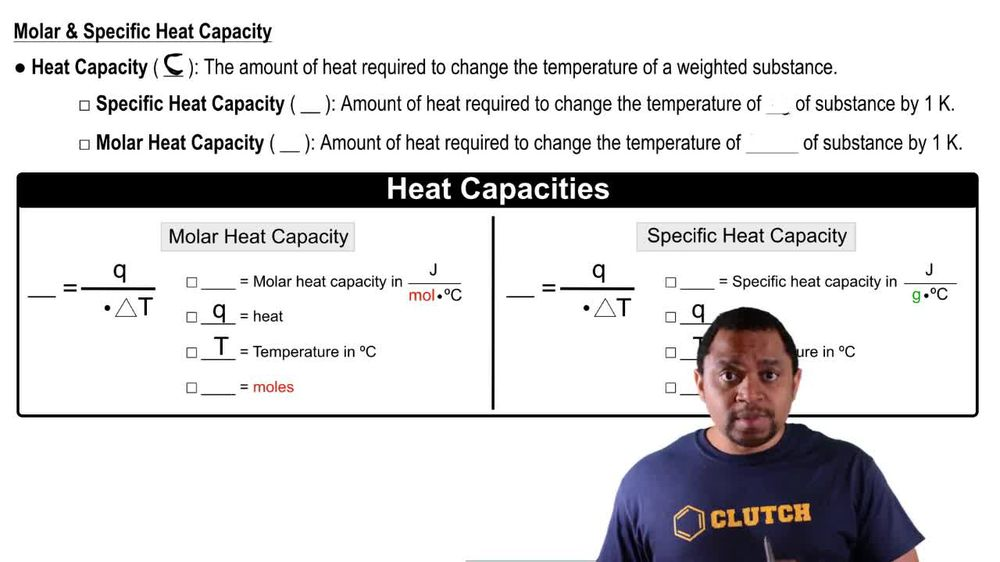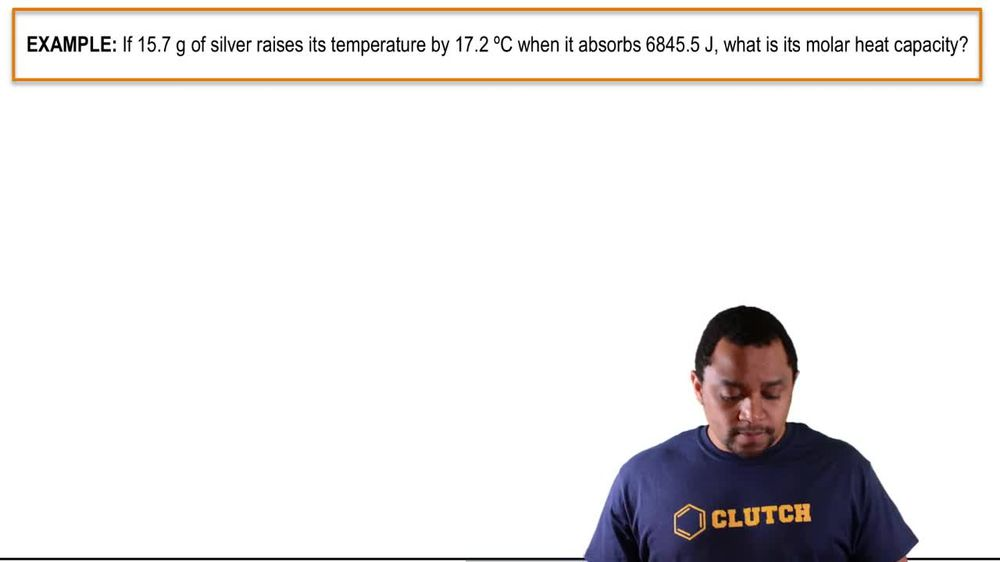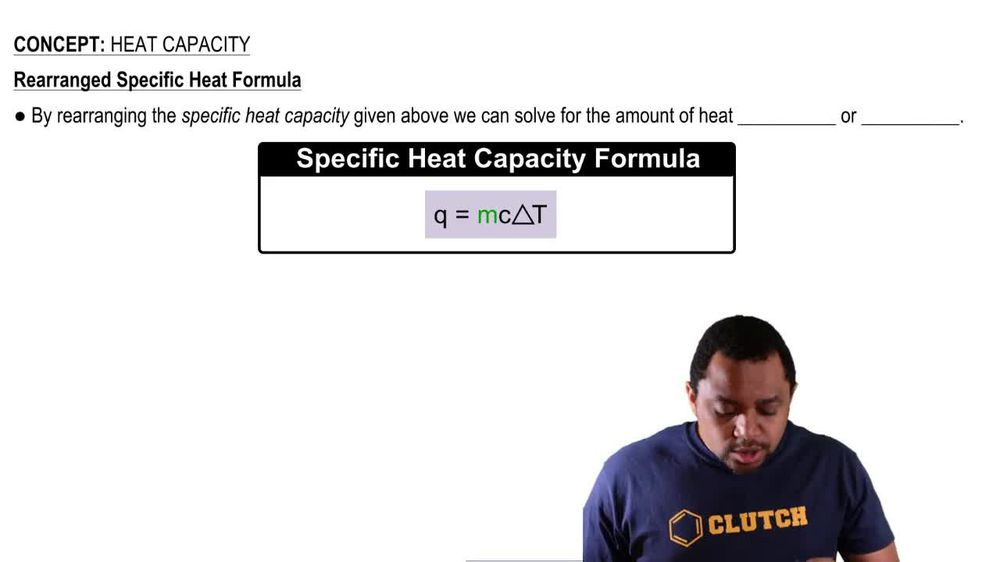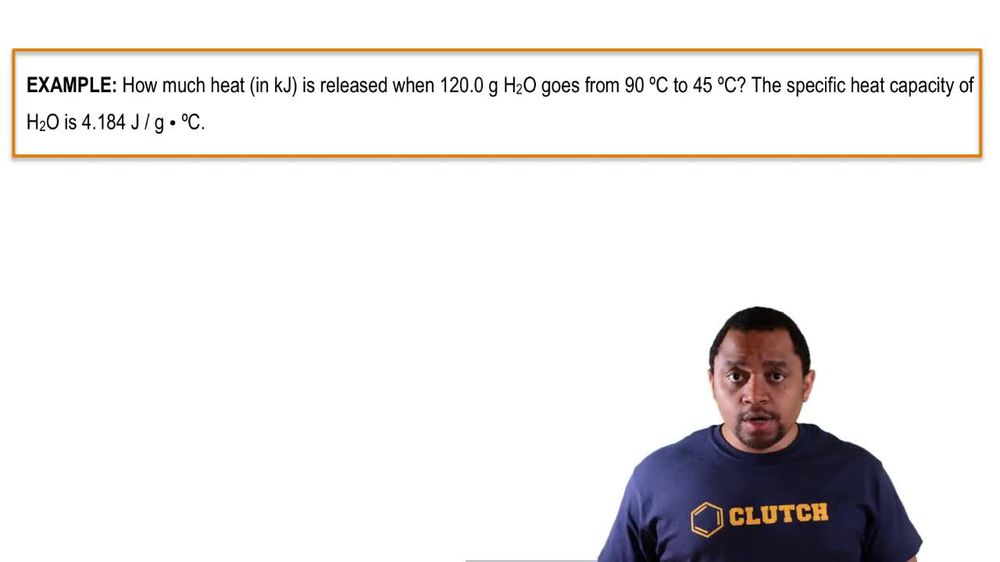Start typing, then use the up and down arrows to select an option from the list.
1. 8. Thermochemistry2. Heat Capacity# Heat Capacity Example 2

by Jules Bruno
171 views
2
0
here. The example says 15.7 g silver raises its temperature by 17.2 degrees Celsius when it absorbs 6845.5 jewels. What is its Mueller? He capacity? So Mueller Heat capacity uses Capital C. It's equal to heat, which is Q divided by moles. End times change in temperature In the question. It says that we're absorbing this much energy. That means that that's a positive. Q. So that's positive. 6145 5 jewels. Next, we need moles, and we already have the change in temperature. They said that the temperature was risen by 17.2 degrees Celsius, so that's already are change in temperature. We need moles. We have here 15.7 g of silver, which is a G. We have to change that. Two moles. So one mole of Silver Ways 107. g according to the periodic table. So that comes out to be 0. Moles of silver. Take those moles and plug it in. So when we do that, that's going to give me my Mueller. He capacity as 2734. Jules Over Moles times, degrees Celsius. If we look at our values, this has three sig figs and this has three sig figs. So I could change this to 2.73 times 10 to the three Jules over moles times degrees Celsius. So that would be the multi capacity for silver under these conditions.